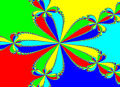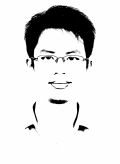# 101師大附中(含計算題)

## 101師大附中(含計算題)

101 師大附中計算證明題，除第三題，兩小題各 5 分外，其餘每題 9 分

1. $$a>0$$, $$b>0$$, $$\theta$$ 銳角，求 $$\frac{a}{\cos\theta}+\frac{b}{\sin\theta}$$ 的最小值。

2. $$\triangle ABC$$ 中， $$G$$ 為重心。直線 $$L$$ 過 $$G$$ 與 $$\overline{AB}$$ 和 $$\overline{BC}$$ 分別交於 $$M$$ 和 $$N$$。

$$\overline{BM}=p\overline{BA}$$, $$\overline{BN}=q\overline{BC}$$，求 $$pq$$ 最小值。

3. $$a_{1}=2$$, $$a_{n+1}=\frac{1}{2}(a_{n}+\frac{1}{a_{n}})$$, for $$n\geq1$$。

(1) 證明 $$\lim\limits_{n\to\infty}a_{n}$$ 存在。

(2) 證明 $$\sum\limits_{n=1}^{\infty}(\frac{a_{n}}{a_{n+1}}-1)$$ 收斂。

4. $$A_{n\times n}=[a_{ij}]$$, $$B_{n\times n}=[b_{ij}]$$，其中 $$b_{ij}=\sum\limits _{k=1}^{n}a_{ik}-a_{ij}$$。$$\det A=a$$, 求 $$\det B$$ (以 $$a$$ 表示)。

5. 求 $$\sum\limits_{n=1}^{10}\left[\frac{x}{n!}\right]=2012$$ 的所有正整數解。

6. 求兩小於 1 的正數，其和小於 1，其積小於 $$\frac{2}{9}$$ 的機率。

7. $$P(a,b)$$ 在 $$x^{2}+y^{2}=5$$ 上，求滿足 $$\log_{2}(b-a)-\log_{8}(3b-5a)=0$$ 的所有點 $$P$$。

PS:感謝一起幫忙回憶的夥伴們^^

【註：weiye 於 2012/05/12, 17:10 加上師大附中公佈的參考答案檔案。】

[ 本帖最後由 tsusy 於 2012-5-15 02:07 PM 編輯 ]

#### 附件101師大附中，答案.doc (49.5 KB)

2012-5-12 17:07, 下載次數: 7905101師大附中.zip (26.07 KB)

2012-5-13 17:27, 下載次數: 7722101師大附中.pdf (362.88 KB)

2012-5-15 14:07, 下載次數: 8430

101.5.15 更新(修正填充1)

TOP

## 回復 1# tsusy 的帖子

[ 本帖最後由 shingjay176 於 2012-5-28 02:43 PM 編輯 ]

#### 附件IMAG0050.jpg (46.49 KB)

2012-5-12 13:52TOP

 tsusy 寸絲發短消息 加為好友 當前離線 3# 大 中 小 發表於 2012-5-12 13:55  只看該作者 想請教是填充 2 ，上面的計算題，只是趁現在還記得，先寫下來而已。 填充 2：$$\triangle ABC$$ 中 $$\overline{AB}=12$$, $$\overline{AC}=8$$, $$D$$ 在 $$\triangle ABC$$ 內部，且 $$\angle ABD =\angle ACD$$, $$\angle ADB$$ 是直角。 $$M$$ 為 $$\overline{BC}$$ 中點，求 $$\overline{DM}$$ 感覺應該是用外接圓，對同弧，在沒做出來，那時候找了一個特例，不過敲鐘後，發現找的特到 $$D$$ 在三角形外面... 網頁方程式編輯 imatheq UID981 帖子1027 閱讀權限10 來自方寸之地 在線時間2946 小時 註冊時間2011-10-10 最後登錄2021-10-17  查看個人網站 查看詳細資料 TOP

## 回復 3# tsusy 的帖子

TOP

6. 求兩小於 1 的正數，其和小於 1，其積小於 2/9的機率。

[(1/6)-(2/9)ln2]=(1/3)+(2/9)ln2

[ 本帖最後由 shiauy 於 2012-5-12 05:56 PM 編輯 ]

#### 附件MWSnap024 2012-05-12, 17_45_13.jpg (27.58 KB)

2012-5-12 17:51TOP

## 回復 4# shingjay176 的帖子[ 本帖最後由 tsusy 於 2012-5-13 03:40 PM 編輯 ]

TOP

#### 附件101 師大附中 計算題2,4,7.pdf (122.93 KB)

2012-5-12 18:41, 下載次數: 7418

TOP

 Yichen 帥氣小賴發短消息 加為好友 當前離線 8# 大 中 小 發表於 2012-5-12 19:37  只看該作者 我比較想要問填充三題目是不是給錯了啊？ 已知∫f(x)  dx = k        0→1 求   ∫  dx ∫  f(x)f(y)dy 0→1         x→1 他的dx是不是應該要放到最後面去才是呢？ [ 本帖最後由 Yichen 於 2012-5-12 07:39 PM 編輯 ] 衛生組長@師大附中 UID1153 帖子3 閱讀權限10 在線時間4 小時 註冊時間2012-5-8 最後登錄2017-10-10  查看詳細資料 TOP

## 回復 8# Yichen 的帖子

TOP

##### 引用:

[ 本帖最後由 Ellipse 於 2012-5-12 10:16 PM 編輯 ]

TOP

﻿